# Orchard

Three-fifths of the orchard are apple trees, one-third of the trees are cherries. The remaining 5 trees are pears. How many trees are in the orchard?

n =  75

### Step-by-step explanation:

3/5 n + 1/3 n + 5 = n

3/5•n + 1/3•n + 5 = n

n = 75

Our simple equation calculator calculates it.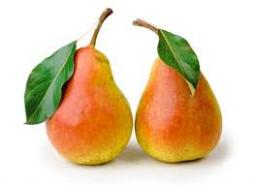Did you find an error or inaccuracy? Feel free to write us. Thank you!Tips to related online calculators
Need help to calculate sum, simplify or multiply fractions? Try our fraction calculator.
Do you have a linear equation or system of equations and looking for its solution? Or do you have a quadratic equation?

## Related math problems and questions:

• Trees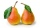Two-fifths of the trees in the orchard are apple trees, one-sixth a pear. The remaining 26 trees are cherries. How many pears are in the orchard?
• Trees - orchardThe 3/5 trees are apple trees and 1/3 of the trees are cherries. The remaining 5 trees are pear trees. How many trees are in the orchard.
• Orchard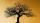3/5 of the trees in the orchard are apples, 1/3 are cherries and the remaining five trees are pear. How many trees are in the orchard?
• An orchard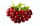In an orchard, there are 28 apple trees, 27 orange trees, and 14 cherry trees. What is the ratio of apple trees to cherry trees?
• There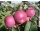There are 32 trees of three species in the orchard. 25% cherries, three eighty pears, others plums. How many plums are in the orchard?
• TreesFrom the total number of trees in the orchard, there are two-fifths pearls and apples are three eighty. The rest of the trees are 9 ceremonial. How many trees are in the set?
• Proportion 3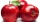For every 8 mango trees in the orchard, there are 4-star apple trees. If there are 1320 trees, how many trees of each kind are there?
• In the orchardIn the orchard, they planted 25 apple trees, 20 pears, 15 plums and 40 marbles. A strong late frost, however, destroyed a fifth of all new trees. Unfortunately, it was all the trees of one kind of fruit. What is the probability that the plums have died ou
• OrchardOne-eighth of the trees in the fruit plant in winter froze, and one-twelfth of damaged disease and pests. Healthy trees remained 152. Is it enough to supply 35 trees to restore the original number of trees in the orchard?
• Trees3/5 trees are apples, cherries are 1/3. 5 trees are pear. How many is the total number of trees?
• PearsThere were pears in the basket, I took two-fifths of them, and left six in the basket. How many pears did I take?
• Trees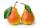In the orchard, 38 apple trees and 20 pear trees grew. How many trees there grow?
• What is 9What is the value of x in the proportion 2 and one-fourth over x = 1 and one-half over 3 and three-fifths? 2 and two-fifths 5 and two-fifths 8 and 1 over 10 12 and 3 over 20
• Orchard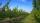10 trees in 5 lines grows in the orchard. How many trees are in the orchard?
• A man 3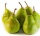A man buys a box of fruits containing 286 fruits out of these 1/2 are apples and the rest are pears. 4/13 of the pears are rotten. He sells the good pears at rupees 4 1/11 each. How much money does he receive on selling the good pears?
• Cut trees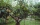There are 1,200 trees in the grove, of which 55 percent are deciduous, the remaining coniferous. Workers cut down 35 percent a) trees, b) deciduous trees, c) coniferous trees. How many trees now have this grove?
• Trees avgThe worker planted 96 trees on Monday, 120 on Tuesday, and 61 trees on Wednesday. How many trees did he plant on Thursday if he averaged 105 trees per day?